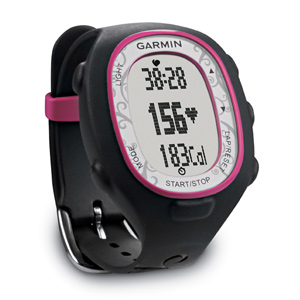# How do heart rate monitors count calories?

Jun 14, 2016

They don’t. They measure heart rate, and that’s it.

#### Explanation:

They estimate calories burned by using an estimated relationship between heart rate (HR) and oxygen uptake ($\text{VO₂}$).

Most people don’t know their $\text{VO₂}$ for a given level of activity, so the manufacturers of heart rate monitors use their own secret formulas.

They try to calculate your rate of caloric expenditure using variables such as gender, heart rate, weight, and age (which are related to $\text{VO₂}$).Typical formulas for calories might be:

For males:

$\text{Calories" = "-55.0969 +0.6309HR + 0.1988W +0.2017A"/4.184 × "60T}$

For females:

$\text{Calories" = "-20.4022 + 0.4472HR -0.1263W + 0.074A"/4.184 × "60T}$

where

$\text{HR = heart rate in beats per minute}$
$\text{W = weight in kilograms}$
$\text{A = age in years}$
$\text{T = exercise duration time in hours}$

If you wore four different watches, you would probably get four different readings.

And just because the watch on your wrist says you burned 1 000 calories, it isn’t necessarily true.

There could be a margin of error of as much as ±50 %.